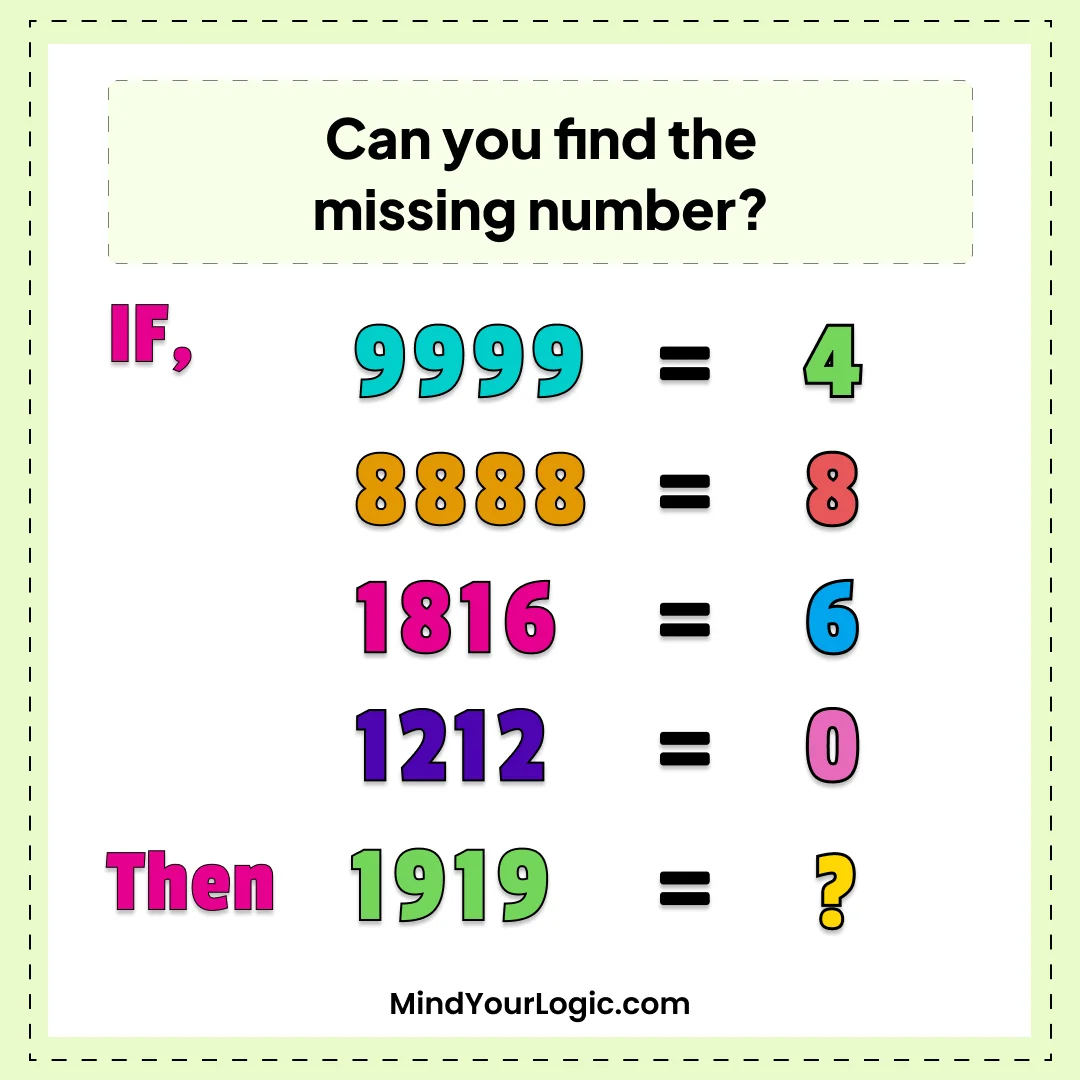# Find The Missing Number - Math Riddle

###### 77.Math Riddles
`Can you find the missing number?`• `2`
```Explanation :

The answer will be 2. Look at how many closed areas there are.
9999 has 4 closed areas (the top of the '9').
8888 has 8 closed areas (the top and bottom parts of 8 and there are no other digits).
1816 has 3 closed areas (top and bottom of 8 and bottom of 6, and it has 2 other digits (3 * 2 = 6)).
1212 has 0 closed areas, (0 * 4 = 0),
Therefore,
1919 has 2 closed areas. (the top of the '9').```